Let us build a simple web-app calculator with a test-first approach and React!

I highly suggest you follow this exercise if you are already familiar with React, and want to also step up your testing game. 🌟

We will approach building a simple app, with only the basic calculation functions - but most importantly - we will follow a Test Driven Developmnet approach.

Test-driven development (TDD) is a development technique where you must first write a test that fails before you write new functional code. TDD is being quickly adopted by agile software developers for development of application source code and is even being adopted by Agile DBAs for database development. (agiledata.org)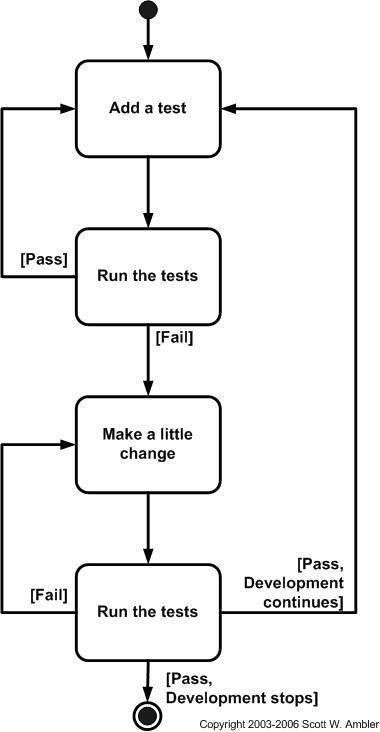Image stolen from agiledata.org.

## Setup

We want a quick setup that provides us with testing already configured for us so we are picking good old `create-react-app` for this. We will also choose the `TypeScript` template. So open a terminal and run:

`npx create-react-app calculator --template typescript`

`cd calculator`

Open an editor. I'll use VS Code:

`code .`

Have two terminal windows open:

• one to run `npm start`

• one to run `npm run test`

## Let's start coding

Start coding you say? Funny. I thought we were doing TDD. Of course, we want to create a failing test first. But where do we start?

Go to `App.test.tsx` , delete the existing test and write:

``````test("renders calculator", () => {
render(<App />);
const calculatorElement = screen.getByText(/calculator/i);
expect(calculatorElement).toBeInTheDocument();
});
``````

And there you go, our terminal that runs the tests should output:

`Tests: 1 failed, 1 total`

Our test just naively checks that we have somewhere in our app the text calculator rendered.

So we will create a `Calculator.tsx` file with:

``````const Calculator = () => <h1>Calculator</h1>;

export default Calculator;
``````

The test still fails. Well.. We are not yet rendering our `Calculator` component. Let's fix that. Go to 'App.tsx':

``````import React from "react";
import "./App.css";
import Calculator from "./Calculator";

function App() {
return (
<div className="App">
<main>
<Calculator />
</main>
</div>
);
}

export default App;
``````

`Tests: 1 passed, 1 total`

• great we passed our first test. Now what?

Do we build the code for the calculator? ❌ Of course not, we write another failing test, now in 'Calculator.test.tsx', to show the calculator numbers:

``````import { render, screen } from "@testing-library/react";
import React from "react";
import Calculator from "./Calculator";

describe("<Calculator />", () => {
it("shows numbers", () => {
render(<Calculator />);
const numbers = [0, 1, 2, 3, 4, 5, 6, 7, 8, 9];

numbers.forEach((n) => {
expect(screen.getByText(n.toString())).toBeInTheDocument();
});
});
});
``````

And in `Calculator.tsx` :

``````+ const numbers = [0, 1, 2, 3, 4, 5, 6, 7, 8, 9];
+ const Calculator = () => {
+   return (
+     <div className="calculator">
<h1>Calculator</h1>
+       {numbers.map((n) => (
+         <button key={n}>{n.toString()}</button>
+       ))}
+     </div>
+   );
+ };

export default Calculator;
``````

Alright, at this point, if we look at the app we have something like: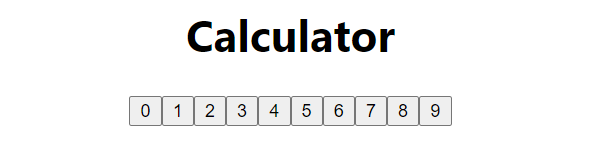## Render rows of numbers

We want to show our numbers in rows: Row 1: [7, 8, 9] Row 2: [4, 5, 6] Row 3: [1, 2, 3] Row 4: 

Hmm.. how do we test that? So in 'Calculator.test.tsx', we could have a new test like:

``````  it("shows 4 rows", () => {
render(<Calculator />);
const rows = screen.getAllByRole("row");

expect(rows).toHaveLength(4);
});
``````

Alright, now that we have the failing test, to pass it:

``````const rows = [[7, 8, 9], [4, 5, 6], [1, 2, 3], ];

const Calculator = () => {
return (
<div className="calculator">
<h1>Calculator</h1>
<div role="grid">
{rows.map((row) => {
return (
<div key={row.toString()} role="row">
{row.map((n) => (
<button key={n}>{n.toString()}</button>
))}
</div>
);
})}
</div>
</div>
);
};

export default Calculator;
``````

Let's also check our app: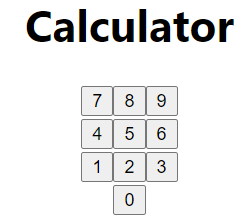## Show calculator operators

Test to show operators:

``````  it("shows calculation operators", () => {
render(<Calculator />);
const calcOperators = ["+", "-", "×", "÷"];

calcOperators.forEach((operator) => {
expect(screen.getByText(operator.toString())).toBeInTheDocument();
});
});
``````

Pass the test:

``````const rows = [[7, 8, 9], [4, 5, 6], [1, 2, 3], ];
+ const calcOperators = ["+", "-", "×", "÷"];
const Calculator = () => {
return (
<div className="calculator">
<h1>Calculator</h1>
<div role="grid">
{rows.map((row) => {
return (
<div key={row.toString()} role="row">
{row.map((n) => (
<button key={n}>{n.toString()}</button>
))}
</div>
);
})}
+       {calcOperators.map((c) => (
+         <button key={c}>{c.toString()}</button>
+       ))}
</div>
</div>
);
};

export default Calculator;
``````

Great! It looks terrible 😅. Don't worry, we will fix the styles later.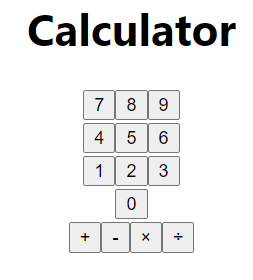## Show an equal sign & clear sign:

Tests:

``````  it("renders equal", () => {
render(<Calculator />);
const equalSign = "=";
expect(screen.getByText(equalSign)).toBeInTheDocument();
});

it("renders clear sign", () => {
render(<Calculator />);
const clear = "C";
expect(screen.getByText(clear)).toBeInTheDocument();
});
``````

Great, 2 tests are failing. To fix:

``````import { Fragment } from "react";

const rows = [[7, 8, 9], [4, 5, 6], [1, 2, 3], ];
const calcOperators = ["+", "-", "×", "÷"];
const equalSign = "=";
const clear = "C";
const Calculator = () => {
return (
<div className="calculator">
<h1>Calculator</h1>
<div role="grid">
{rows.map((row, i) => {
return (
<Fragment key={row.toString()}>
<div role="row">
{i === 3 && <button>{clear}</button>}
{row.map((n) => (
<button key={n}>{n}</button>
))}
{i === 3 && <button>{equalSign}</button>}
</div>
</Fragment>
);
})}
{calcOperators.map((c) => (
<button key={c}>{c.toString()}</button>
))}
</div>
</div>
);
};

export default Calculator;
``````

## Show an input for values to be calculated

Test:

``````  it("renders an input", () => {
render(<Calculator />);
expect(screen.getByPlaceholderText("calculate")).toBeInTheDocument();
});
``````

We always want this input to be disabled, so we will also add a test for that:

``````  it("renders an input disabled", () => {
render(<Calculator />);
expect(screen.getByPlaceholderText("calculate")).toBeDisabled();
});
``````

Implement the input:

``````+ import { Fragment, useState } from "react";

const rows = [[7, 8, 9], [4, 5, 6], [1, 2, 3], ];
const calcOperators = ["+", "-", "×", "÷"];
const equalSign = "=";
const clear = "C";
const Calculator = () => {
+  const [value, setValue] = useState("");
return (
<div className="calculator">
<h1>Calculator</h1>
+     <input
+      type="text"
+      defaultValue={value}
+      placeholder="calculate"
+      disabled
+     />
<div role="grid">
{rows.map((row, i) => {
return (
<Fragment key={row.toString()}>
<div role="row">
{i === 3 && <button>{clear}</button>}
{row.map((n) => (
<button key={n}>{n}</button>
))}
{i === 3 && <button>{equalSign}</button>}
</div>
</Fragment>
);
})}
{calcOperators.map((c) => (
<button key={c}>{c.toString()}</button>
))}
</div>
</div>
);
};

export default Calculator;
``````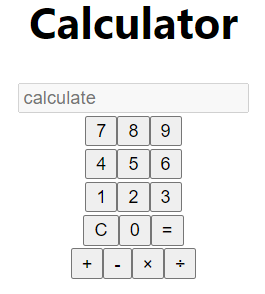## Make it display the user's inputs

Tests:

``````  it("displays users inputs", async () => {
render(<Calculator />);
const one = screen.getByText("1");
const two = screen.getByText("2");
const plus = screen.getByText("+");
fireEvent.click(one);
fireEvent.click(plus);
fireEvent.click(two);

const result = await screen.findByPlaceholderText("calculate");
// @ts-ignore
expect(result.value).toBe("1+2");
});

it("displays multiple users inputs", async () => {
render(<Calculator />);
const one = screen.getByText("1");
const two = screen.getByText("2");
const three = screen.getByText("3");
const five = screen.getByText("5");
const divide = screen.getByText("÷");
const mul = screen.getByText("×");
const minus = screen.getByText("-");
fireEvent.click(three);
fireEvent.click(mul);
fireEvent.click(two);
fireEvent.click(minus);
fireEvent.click(one);
fireEvent.click(divide);
fireEvent.click(five);

const result = await screen.findByPlaceholderText("calculate");
// @ts-ignore
expect(result.value).toBe("3×2-1÷5");
});
``````

Pass the tests:

``````               <div role="row">
{i === 3 && <button>{clear}</button>}
{row.map((n) => (
-                  <button key={n}>{n}</button>
+                  <button
+                    onClick={() => setValue(value.concat(n.toString()))}
+                    key={n}
+                  >
+                    {n}
+                  </button>
))}
{i === 3 && <button>{equalSign}</button>}
</div>
``````
``````         {calcOperators.map((c) => (
-          <button key={c}>{c.toString()}</button>
+          <button onClick={() => setValue(value.concat(c))} key={c}>
+            {c.toString()}
+          </button>
))}
``````

## Can it calculate?

Alright, so up until now we just wrote some tests to check if our calculator displays the right stuff, but let us write some tests for it to actually calculate something:

``````  it("calculate based on users inputs", async () => {
render(<Calculator />);
const one = screen.getByText("1");
const two = screen.getByText("2");
const plus = screen.getByText("+");
const equal = screen.getByText("=");
fireEvent.click(one);
fireEvent.click(plus);
fireEvent.click(two);
fireEvent.click(equal);

const result = await screen.findByPlaceholderText("calculate");

expect(
(result as HTMLElement & {
value: string;
}).value
).toBe("3");
});

it("calculate based on multiple users inputs", async () => {
render(<Calculator />);
const one = screen.getByText("1");
const two = screen.getByText("2");
const three = screen.getByText("3");
const five = screen.getByText("5");
const divide = screen.getByText("÷");
const mul = screen.getByText("×");
const minus = screen.getByText("-");
const equal = screen.getByText("=");

fireEvent.click(three);
fireEvent.click(mul);
fireEvent.click(two);
fireEvent.click(minus);
fireEvent.click(one);
fireEvent.click(divide);
fireEvent.click(five);
fireEvent.click(equal);

const result = await screen.findByPlaceholderText("calculate");
expect(
(result as HTMLElement & {
value: string;
}).value
).toBe("5.8");
});
``````

Notice in our second test we also check that the operations are executed in the correct order:

`3*2-1÷5 = 6-0.2 = 5.8`

And, let us make this pass. At this stage, we can use the unsafe `eval` function, which we will refactor later. Remember, we only need to pass the tests. We can always write a test to propose why our current implementation is not ok.

``````const rows = [[7, 8, 9], [4, 5, 6], [1, 2, 3], ];
const calcOperators = ["+", "-", "×", "÷"];
const equalSign = "=";
const clear = "C";
+
+const calculateExpression = (expression: string) => {
+  const mulRegex = /×/g;
+  const divRegex = /÷/g;
+
+  const toEvaluate = expression.replace(mulRegex, "*").replace(divRegex, "/");
+
+  // todo - refactor eval later
+  const result = eval(toEvaluate);
+  return result;
+};
+
const Calculator = () => {
const [value, setValue] = useState("");
+
+  const calculate = () => {
+    const results = calculateExpression(value);
+    setValue(results);
+  };
+
return (
<div className="calculator">
<h1>Calculator</h1>
@@ -29,7 +47,7 @@ const Calculator = () => {
{n}
</button>
))}
-                {i === 3 && <button>{equalSign}</button>}
+                {i === 3 && <button onClick={calculate}>{equalSign}</button>}
</div>
</Fragment>
);
``````

## Can use clear button

The test:

``````  it("can clear results", async () => {
render(<Calculator />);
const one = screen.getByText("1");
const two = screen.getByText("2");
const plus = screen.getByText("+");
const clear = screen.getByText("C");
fireEvent.click(one);
fireEvent.click(plus);
fireEvent.click(two);

fireEvent.click(clear);

const result = await screen.findByPlaceholderText("calculate");
expect(
(result as HTMLElement & {
value: string;
}).value
).toBe("");
});
``````

Easy:

``````const Calculator = () => {
setValue(results);
};
+
+  const clearValue = () => setValue("");
+
return (
<div className="calculator">
<h1>Calculator</h1>
@@ -38,7 +40,7 @@ const Calculator = () => {
return (
<Fragment key={row.toString()}>
<div role="row">
-                {i === 3 && <button>{clear}</button>}
+                {i === 3 && <button onClick={clearValue}>{clear}</button>}
{row.map((n) => (
``````

## Back to calculating stuff

Alright, so at this point, we maybe want to test more scenarios for the calculate function. So I think it makes more sense to write those tests directly on the `calculateExpression` function.

So we will export it and write some extra tests:

``````describe("calculateExpression", () => {
it("correctly computes for 2 numbers with +", () => {
expect(calculateExpression("1+1")).toBe(2);
expect(calculateExpression("10+10")).toBe(20);
expect(calculateExpression("11+345")).toBe(356);
});

it("correctly substracts 2 numbers", () => {
expect(calculateExpression("1-1")).toBe(0);
expect(calculateExpression("10-1")).toBe(9);
expect(calculateExpression("11-12")).toBe(-1);
});

it("correctly multiples 2 numbers", () => {
expect(calculateExpression("1×1")).toBe(1);
expect(calculateExpression("10×0")).toBe(0);
expect(calculateExpression("11×-12")).toBe(-132);
});

it("correctly divides 2 numbers", () => {
expect(calculateExpression("1÷1")).toBe(1);
expect(calculateExpression("10÷2")).toBe(5);
expect(calculateExpression("144÷12")).toBe(12);
});

it("division by 0 returns 0 and logs exception", () => {
const errorSpy = jest.spyOn(console, "error");
expect(calculateExpression("1÷0")).toBe(undefined);
expect(errorSpy).toHaveBeenCalledTimes(1);
});
});
``````

Our tests still pass, except for the one with the division by 0. That's good. Let's fix that.

``````-const calculateExpression = (expression: string) => {
+export const calculateExpression = (expression: string) => {
const mulRegex = /×/g;
const divRegex = /÷/g;
+  const divideByZero = /\/0/g;

const toEvaluate = expression.replace(mulRegex, "*").replace(divRegex, "/");

-  // todo - refactor eval later
-  const result = eval(toEvaluate);
-  return result;
+  try {
+    if (divideByZero.test(toEvaluate)) {
+      throw new Error("Can not divide by 0!");
+    }
+
+    // todo - refactor eval later
+    const result = eval(toEvaluate);
+
+    return result;
+  } catch (err) {
+    console.error(err);
+    return undefined;
+  }
};
``````

Ok, more tests for some extra cases:

``````  it("handles multiple operations", () => {
expect(calculateExpression("1÷1×2×2+3×22")).toBe(70);
});

it("handles trailing operator", () => {
expect(calculateExpression("1÷1×2×2+3×22+")).toBe(70);
});

it("handles empty expression", () => {
expect(calculateExpression("")).toBe(undefined);
});
``````

## Watercooler 🌊

Alright, if you made it until here, congrats! 🙌 You are learning how to write code in a TDD way.

Please notice, at this point, the mentality is to add tests and see what tests fail. Maybe some will pass, some will fail, but we want to make sure we have a test-first approach and we are careful with the quality of the tests. If our tests are good, and they all pass, the app will perform well.

So let us fix the 2 failing tests we have now:

`````` const clear = "C";

+const getLastChar = (str: string) => (str.length ? str[str.length - 1] : "");
+const isNumber = (str: string) => !isNaN(Number(str));
+
export const calculateExpression = (expression: string) => {
+  if (!expression || expression.length === 0) {
+    return;
+  }
+
const mulRegex = /×/g;
const divRegex = /÷/g;
const divideByZero = /\/0/g;

-  const toEvaluate = expression.replace(mulRegex, "*").replace(divRegex, "/");
+  let toEvaluate = expression.replace(mulRegex, "*").replace(divRegex, "/");

try {
if (divideByZero.test(toEvaluate)) {
throw new Error("Can not divide by 0!");
}
+
+    const lastCharaterIsNumber = isNumber(getLastChar(toEvaluate));
+
+    if (!lastCharaterIsNumber) {
+      toEvaluate = toEvaluate.slice(0, -1);
+    }
+
``````

## Get rid of eval

Remember when we said we will change eval to something else. Yes, we want to avoid it as our linter and our common sense dictates we should not use it.

Luckily there is a package that does exactly what we want. Pass a string as an expression and safely evaluate it:

`yarn add mathjs @types/mathjs`

``````+import { evaluate } from "mathjs";
``````
``````-    // todo - refactor eval later
-    const result = eval(toEvaluate);
+    const result = evaluate(toEvaluate);
``````

What... our tests still pass? Cool!

## Style the app

But the app is really ugly.. Let's fix that.

First, let us declare some variables in the `index.css` :

``````:root {
--theme-color-dark-10: # 006ba1;
--theme-color-dark-20: # 005a87;
--theme-color-background: # fed800;
}
``````

In the body we will just add the background color and leave the rest of the styles as they are:

`````` body {
+  background-color: var(--theme-color-background);
``````

We will need to add a bit more structure to our `Calculator.tsx` :

``````import { Fragment, useState } from "react";
import { evaluate } from "mathjs";
import "./Calculator.css";

const rows = [[7, 8, 9], [4, 5, 6], [1, 2, 3], ];
const calcOperators = ["+", "-", "×", "÷"];
const equalSign = "=";
const clear = "C";

const getLastChar = (str: string) => (str.length ? str[str.length - 1] : "");
const isNumber = (str: string) => !isNaN(Number(str));

export const calculateExpression = (expression: string) => {
if (!expression || expression.length === 0) {
return;
}

const mulRegex = /×/g;
const divRegex = /÷/g;
const divideByZero = /\/0/g;

let toEvaluate = expression.replace(mulRegex, "*").replace(divRegex, "/");

try {
if (divideByZero.test(toEvaluate)) {
throw new Error("Can not divide by 0!");
}

const lastCharaterIsNumber = isNumber(getLastChar(toEvaluate));

if (!lastCharaterIsNumber) {
toEvaluate = toEvaluate.slice(0, -1);
}

const result = evaluate(toEvaluate);

return result;
} catch (err) {
console.error(err);
return undefined;
}
};

const Calculator = () => {
const [value, setValue] = useState("");

const calculate = () => {
const results = calculateExpression(value);
setValue(results);
};

const clearValue = () => setValue("");

return (
<div className="calculator">
<h1>Calculator</h1>
<input
type="text"
defaultValue={value}
placeholder="calculate"
disabled
/>
<div className="calculator-buttons-container">
<div role="grid">
{rows.map((row, i) => {
return (
<Fragment key={row.toString()}>
<div role="row">
{i === 3 && <button onClick={clearValue}>{clear}</button>}
{row.map((n) => (
<button
key={n}
onClick={() => setValue(value.concat(n.toString()))}
>
{n}
</button>
))}
{i === 3 && <button onClick={calculate}>{equalSign}</button>}
</div>
</Fragment>
);
})}
</div>
<div className="calculator-operators">
{calcOperators.map((c) => (
<button key={c} onClick={() => setValue(value.concat(c))}>
{c.toString()}
</button>
))}
</div>
</div>
</div>
);
};

export default Calculator;
``````

We will also add a `Calculator.css` file, with:

``````.calculator > h1 {
color: var(--theme-color-dark-20);
text-transform: uppercase;
}

.calculator input {
height: 2.5rem;
width: 13rem;
border: 1px solid white;
margin: 0.3rem 0.3rem 1.5rem 0.3rem;
font-size: 1.5rem;
color: var(--theme-color-dark-20);
box-shadow: 8px 8px 5px -7px var(--theme-color-dark-10);
}

.calculator button {
width: 3.5rem;
height: 3.5rem;
font-size: 1.5rem;
color: var(--theme-color-dark-20);
}

.calculator-buttons-container {
display: flex;
align-items: center;
justify-content: center;
}

.calculator-operators {
display: flex;
flex-direction: column;
}
``````

Looks better.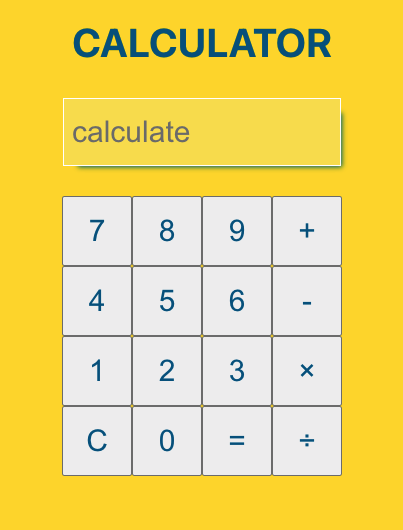## Conclusions

I want to stop here - still, the app has some bugs and things that can be fixed.

If you are up for it, fix them in a TDD style 🔥. Also, if you can add a new feature like keyboard support, in a TDD way, let me know on Twitter where I post daily new content about programming.

Here's the repo for this coding exercise.

Until next time, let me know, do you practice TDD?

This post is also available on DEV.